## 31 August 2021

### Ital-Mil 1200Bd/800 FSK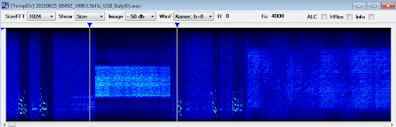Short Ital-Mil 1200Bd/800 FSK used before STANAG-4285/HDLC QUEDRE . The bitstream after demodulation exhibits a 232-bit period length and a bit more strutured patterns after differential decoding (figure 1).Figure 1 - demodulated bitstream before (upper) and after differential decoding

Reshaping the differential decoded stream it's interesting notice the 8-bit framing in figure 2 with one bit always set to "1" (or "0" according the polarity). The same 8-bit framing can be seen in a 46.6 ms window of the SA raster: 46.6ms @1200Bd -> 56 bit -> 7 x 8-bit frames (figure 3). As a guess, the 232-bit period could correspond to a 29-byte protocol data unit carryng almost the same contents.Figure 2 - 8-bit framing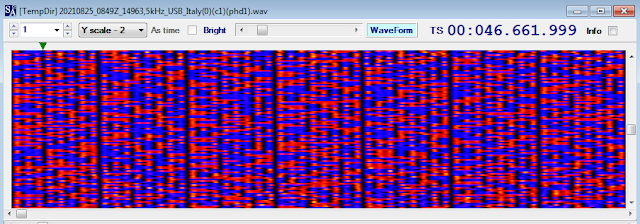Figure 3 (notice the change of polarity each 8 bit)

https://disk.yandex.com/d/xj5xo3vrZB00Jg

## 30 August 2021

### MFSK-16 100Bd/100 transmissions, likely adaptive tests

Interesting MFSK-16 100Bd/100 transmissions spotted on 15810.0 KHz (cf) on August 27th, in the following days I tried several times to monitor that frequency, and more generally the 15 MHz band, but I never had the opportunity to hear them again.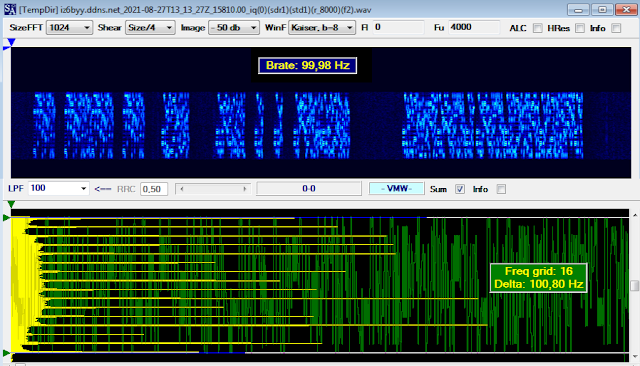Figure 1 - 100Bd/100Hz signal

Some salient features are the presence of series of bursts that have the same pattern and therefore carry the same data, I do not think they act as "calls" but rather idle signaling or test patterns. A second curious feature is a kind of FSK modulations (with different shifts but basically reversals) which are apparently inserted without a precise pattern or timing (figure 2).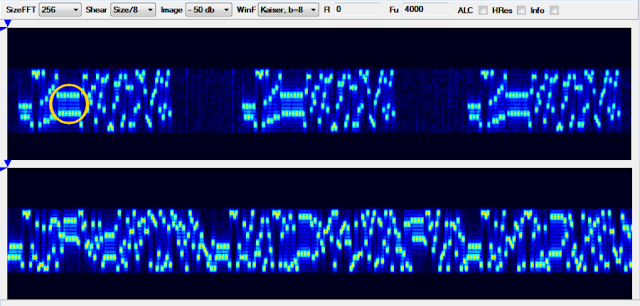Figure 2 - FSK insertions

After demodulation, a plain hex to bin conversion (mapping: 0 -> 0000 ... F -> 1111) doesn't provide much help other than a fairly well defined 438-bit period (figure 3). Likely the signal is transmitted from Ukraine and it's related to test of an adaptive waveform, as my friend KarapuZ say.Figure 3 - 438 bit length period after demodulation

Something regular appears when syncing on 1111111111110111100, but it's only speculating (figure 4).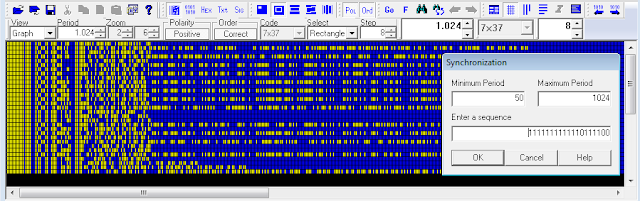Figure 4

https://disk.yandex.com/d/03HlBlUHFTQtKw

## 17 August 2021

### 188-110A, D1 D2 patterns and interleaved blocks boundaries (hardware implementation)

As well as to have some fun with ICs, I wanted to implement a part of the 188-110 modem to definitively understand the relationship between the periodicity of its bit stream and the lengths of the scrambler/interleaver (as indeed already discussed in other blog posts). The "core" is the data sequence randomizing generator: a 12-bit LFSR (Linear Feed Shift Register) with the functional "one-to-many" configuration and described by the polynomyal x^12+x^6+x^4+x+1. I implemented that LFSR by using twelve D-type flip-flop and three XOR gates, plus three 8-bit serial-to-parallel shift registers to manage the LFSR initial state; its operation, and other 188-110A modem functions such as clock, symbols formation and scrambler, are controlled by an Arduino board. The part more strictly related to the circuit (logic and electronics) is illustrated in the second part of the post.

That set of wires & ICs works great and my tests mainly concerned the data rate of 2400 bps: at that speed the framing has a duration of  48-symbols: 32 symbols in the UnKnown data position followed by a 16 symbols in the Known data (probes) position. As expected, the period of the bit stream is 480-symbol/1440-bit length (ie 10 frames) and matches exactly  3 runs of the sequence randomizing generator (reset'd every 160 symbol): regardless of the interleaver block length (figure 1).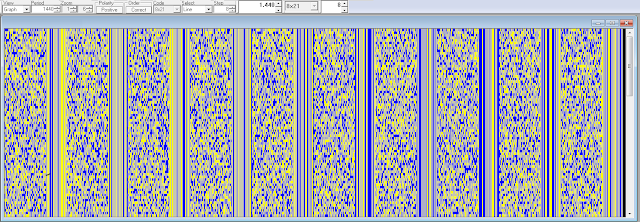Fig. 1 - 1440-bit/480-symbol period of the Arduino bit stream

However, there is still an uncertainty about the length of one interleaver block (short or long), or, better, about how it is indicated.
According to MIL 188-110 Standard, when the two Known symbol patterns preceding the transmission of each new interleaver block are transmitted, the symbols of these two Known patterns shall be set to Dl and D2 values, respectively, as defined in table XV of the standard. These two particular symbol patterns are indicated in figures 2,3 respectively for a real-world signal and the Arduino signal (both are 2400bps/Short).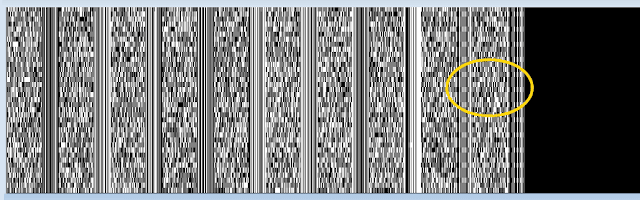Fig. 2 - D1 D2 patterns in a real-world bit streamFig. 3 - D1 D2 patterns in the Arduino bit stream

As you may check, each three rows the patterns of the last two probes exhibit the discontinuity due to the D1 and D2 values: therefore, since that three rows of the 480-symbols period contain 3×10 = 30 frames, the block length consists of 30×32 = 960 tribit symbols for the short interleave setting. The measured value is in constrast with what indicated in the standard; indeed, quoting MIL 188-110B #5.3.2.3.7.1.2,  "[...] The block length shall be 1440 tribit channel symbols for short interleave setting and 11520 tribit channels symbols for the long interleave setting".
You will get 1440 symbols if you consider also the probe symbols, ie if you compute 30×48 instead of 30×32... but the probe symbols are not interleaved, ie they are not fetched from the interleaver matrix! On the other hand, the short interleaver matrix for 2400 bps consists of 40 rows and 72 columns, ie 2880 bit that just will form 960 tribit symbols: this way, one interleaver block coincides with the dimensions of the correspondent intereleaver matrix. A similar calculation can be verified for the long interleave settings.

So, in my opinion, it seems that when 188-110 Sandard talks about the length of one interleaver block, it refers to all(!) the bit that compose UnKnown and Kown data symbols (indeed it talk generically of "channel" symbols) and not to the bit which are actually fetched from the interleaver matrix and that consequently will be used to form the UnKnown data symbols only (as it would seem more logical to me).

Notice that if the sequence randomizing generator is reset to 0xBAD after 80 transmit symbols, the resulting bit stream is 240-symbol/720-bit length (5 frames), ie just the half of the normal operations (as expected!).Fig. 4 - 720-bit period (LFSR reset'd after 80 transmit symbols)

Arduino part
As said above, the sequence randomizing generator is a 12-bit LFSR that I implemented by using 12 D-type flip-flops (6 x 74HC74) and 3 XOR gates (74HC86) used for the feedback path. Since the LFSR shall be preload to the initial seed 0xBAD (101110101101), we need to control the async set/reset inputs of the 12 flip-flops, therefore we need to manage 24 pins from the Arduino board and probably we do not have such number of ports available on the board. The idea is to use a serial-in parallel-out shift register (74HC595) to control 8 lines at a time, so link three registers together will give us the chance to get 24 parallel lines by sending 3 bytes on a serial pin of the Arduino board; that way, each 8-bit shift register will drive the set/reset inputs of four flip-flops (figure 5).Fig. 5 -  three 8-bit shift register used to manage twelve D-type flip-flop

The connections for wiring the preload part are shown in figure 6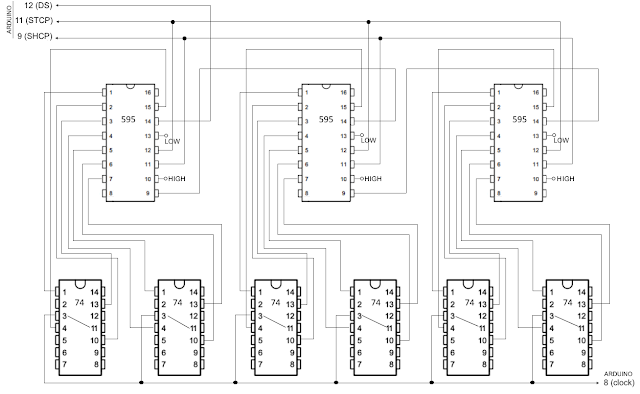Fig. 6
188-110 Standard suggests to implement the sequence randomizing generator using a 12-bit LFSR in the "one-to-many" configuration, ie the most-significant bit is fed back directly into the least significant bit, and is also individually XORed with the other bits 6,4,1 (below in Figure 5). The connections for wiring are shown in figure 7.
https://disk.yandex.com/d/TJvzhdThgCwClA

74HC74 is a dual positive edge triggered D-type flip-flop with individual data (nD), clock (nCP) inputs and asynchronous set (nSD) and reset (nRD) inputs.

74HC86 is a quad 2-input EXCLUSIVE-OR gate.

74HC595 is an 8-bit serial-in/serial or parallel-out shift register with a storage register and 3-state outputs. Both the shift and storage register have separate clocks. The device features a serial input (DS) and a serial output (Q7S) to enable cascading.pinning of the used ICs

Hardware (Arduino + breadboard) and software implement the red-circled parts of the 188-110A modem:

## 6 August 2021

### another approach to the analysis of unresolved sync sequences

The pseudo-random binary sequences (PRBS, also referred to as pseudo-random bit sequences) are largely used in telecommunication (data scrambling, sync sequences, field delimiter markers, jamming, stream ciphers, bit-error rate tests, ...) and are usually generated by physical Linear Feedback Shift Registers (LFSRs), or their software implementations. Sometimes it may happen to come across sync sequences or particular patterns of which it is not possible to trace back to the generator polynomial and therefore to the structure of the used LFSR. This happens because most of the time PRBS are drained from the serial output of the LFSR and therefore the search for the generator polynomial takes place brute-force and "directly" on the pattern being analyzed.Fig. 1 - x^4+x^3+1 LFSR with serial output

But it could happen that the pseudo-random sequence is instead formed by taking the outputs (the states) of all the stages that compose the LFSR, let's say a parallel-output PRBS as an alternative counterpart to the serial-output one.Fig. 2 - x^4+x^3+1 LFSR with parallel outputs

In the first case (serial-output PRBS) the sequence has a length (or period T) of 15 bit while in the second case (parallel-output PRBS) the sequence has a period  of 15×4=60 bit length. Analyzing the latter as if it were a serial-output one could produce not significant or even misleading results, as shown in figure 3.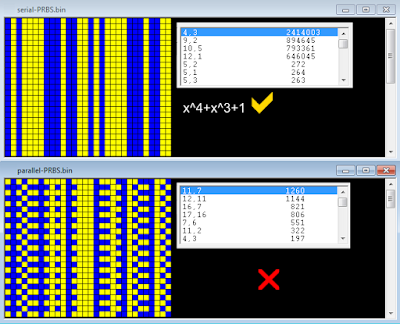Fig. 3 - results after analyzing the serial and parallel output of the same LFSR (x^4+x^3+1)

What we need is establish the number of stages that compose the unknown LFSR, after which a single stage can be isolated and its (serial) output sequence analyzed. Well, assuming T is the period of the pattern, this one shall be reshaped to a c-columns array where c = T/((2^n)-1), where n = (2,3,4,5,6,7...).  After reshaped the original pattern, the search for the generator polynomial can be performed on any of the c columns of that array.  In a few words, we have to found the valor the period T in table below (if any) and then reshape the pattern according to the corrispondent value of c (ie, the number of the stages of the LFSR):

In case of the x^4+x^3+1 LFSR mentioned above, its parallel output has a period length of 60 bit and  is divisible by 15, giving 4 as the number of the stages: the polynomial is then easily verified after reshaping the 60-bit pattern to a 4-bit array (figure 4). Notice that the the length of the serial-output PRBS is just equal to 15.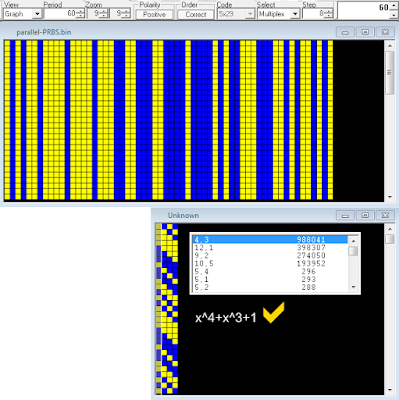Fig. 4

Other examples are shown below:

Obviously, the above is valid only for certain lengths of the period T and it is not certain that it is also valid for generator polynomials that are not primitive, i.e. they do not generate sequences of maximum length . Moreover, it could be argued that a multiplexer is needed to send the parallel-output PRBS, but the whole sequence could also be stored and then pre-loaded and added to the bitstream as many times as needed, or it could happen that only a segment of a parallel-output PRBS could be used.

I am definitely not a "mathematician" but I think that sometimes the above method, though a bit raw, can be useful to unearth the LFSR which is hidden behind particular sequences.

## 3 August 2021

### use PuTTY as Arduino monitor for LFSR circuits

Given the lack of really interesting signals on air, at least these days or no luck in hunting, I am playing with the semi-hardware (1) implementation of pseudo-random sequence generators: a way to spend these hot afternoons combining electronics and signal analysis. Waiting for the delivery of the 74xx595 chips, necessary for the 188-110A sequence generator, I wanted to implement a version of the LFSR (Linear Feedback Shift Register) described by the polynomial x^4+x+1 and verify - even if there was no need - its binary sequence.

So, why a post title about PuTTY? I needed to save the bit stream produced by the circuit for its subsequent graphical representation but unfortunately the Arduino' console (embedded in its development environment) does not allow the content to be saved unless you stop the process and use the copy & paste method, a workaround not always effective in case of output that cause scrolling screen: using PuTTy is just what you need. "Putty" is a free SSH and telnet client for Windows and if it's not yet part of your resources you can quickly download and install it from its site . After launched, select the right serial port number and speed as indicated by Arduino then on the session tab select the printable output and the path of the output file (figure 1): that's all, power-on your circuit and connect Putty, you do not have to run the Arduino environment to get a monitor.Fig. 1 - configuring PuTTY for use as Arduino' monitorFig. 2 - PuTTY at work

Back to the LFSR circuit (four D-type Flip Flop and one XOR gate), its logic is quite simple and does not require much explanation even if I'm not really an artist in wiring the bredboards (figs. 3,4). Just notice that I use the Arduino MEGA 2560 so if you do not use such board you will have to change the input/output pins 6 (preset/clear), 8 (clock), 10 (Q1), 11 (Q2), 12 (Q3), 13 (Q4) by editing the sketch  according to your choices. Mind you that the clocking speed is exaggeratedly low to allow both the set of  the initial state of the registers (see below) and an easier reading of the monitor.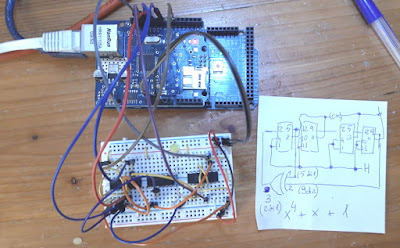Fig. 3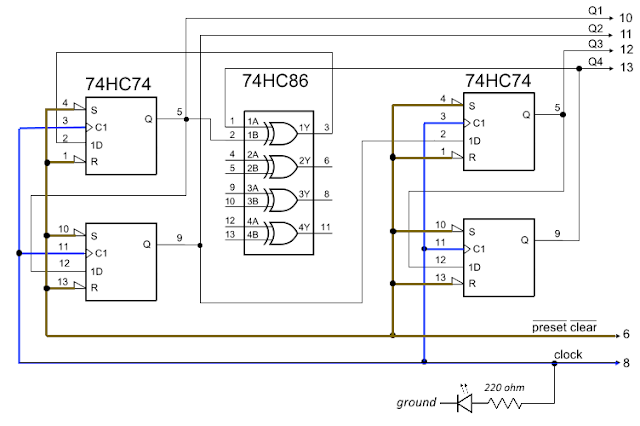Fig. 4

As said, the LFSR is described by the primitive polynomial x^4+x+1, i.e. its sequence is termed as m-sequence (maximum-length sequence) and has a length T = 2^n -1, where n is the number of the used registers (also termed as "stages"); in this case the LFSR employes four registers thus produces a 15-bit length sequence that repeats itself over time. Storing the "state" of each register (outputs Q1, Q2, Q3, Q4) we get 4 × 15 = 60-bit length pattern (figure 6).
Notice that the 0000 state is never crossed since in that state the sequence simply does not start, the registers are as stuck in that state; therefore, the 0000 state is never used as initial state (or "seed") of the LFSR. To start the state' cycles and set the seed to 1000, you will need to keep pin2 HIGH just for a clock positive transition, ie simply wire pin 2 - the data input of the first register - to the +5 V line of the bredboard, clock pulses are monitored by the LED in figure 4.

Obviously, the same sequence will be obtained by simulating the LFSR using an Octave script (LFSRv2.m)  or the Logic Simulator App   or the LFSR Testbench too : you have many tools at your disposal.Fig. 5 - LFSR states output logged by PuTTY and states output produced by Octave script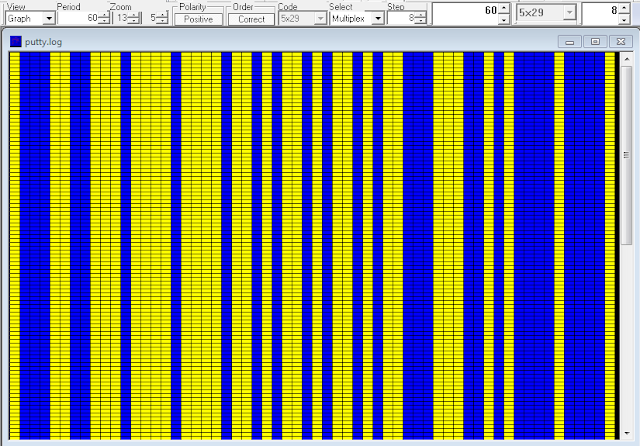Fig. 6 - the 60-bit patterns generated by the four states of the LFSR

The sequences in figures 5-6 are obtained implementing the "many-to-one" feedback structure but you could also implement its "one-to-many" counterpart (2): in which case the sequence of the states will be slightly different (!) though the initial states are the same (figure 7).Fig. 7 - many-to-one and one-to-many feedback structures for x^4+x+1 LFSR

«A way to spend these hot afternoons combining electronics and signal analysis» I said... yep, but the beach is much more better!

(1) semi-hardware since some features such as the clock are software implemented

(2) in "one-to-many" configuration there is never more than one level of combinational logic in the feedback path, irrespective of the number of taps being employed in the traditional "many-to-one" configuration (increasing the levels of logic in the combinational feedback path can negatively impact the maximum clocking frequency).

 https://www.putty.org
 https://disk.yandex.com/d/U4rXrZ7JWWgAGQ
 LFSRv2.m Octave script https://disk.yandex.com/d/jOX64f8u19jYFw
 Logic Simulator project https://disk.yandex.com/d/UcLU_1eAwtubXA
 Logic Simulator Apk https://logic-circuit-simulator-pro.it.aptoide.com/app
 https://disk.yandex.com/d/hMe696WAWjth8Q